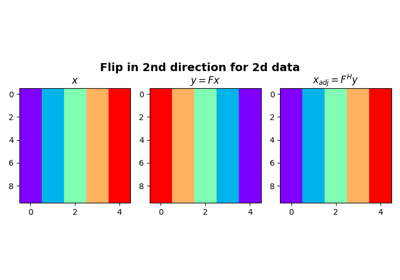# pylops.Flip¶

class pylops.Flip(dims, axis=-1, dtype='float64', name='F')[source]

Flip along an axis.

Flip a multi-dimensional array along axis.

Parameters: dims : Number of samples for each dimension axis : int, optional New in version 2.0.0. Axis along which model is flipped. dtype : str, optional Type of elements in input array. name : str, optional New in version 2.0.0. Name of operator (to be used by pylops.utils.describe.describe)

Notes

The Flip operator flips the input model (and data) along any chosen direction. For simplicity, given a one dimensional array, in forward mode this is equivalent to:

$y[i] = x[N-1-i] \quad \forall i=0,1,2,\ldots,N-1$

where $$N$$ is the dimension of the input model along axis. As this operator is self-adjoint, $$x$$ and $$y$$ in the equation above are simply swapped in adjoint mode.

Attributes: shape : tuple Operator shape explicit : bool Operator contains a matrix that can be solved explicitly (True) or not (False)

Methods

 __init__(dims[, axis, dtype, name]) Initialize this LinearOperator. adjoint() Hermitian adjoint. apply_columns(cols) Apply subset of columns of operator cond([uselobpcg]) Condition number of linear operator. conj() Complex conjugate operator div(y[, niter, densesolver]) Solve the linear problem $$\mathbf{y}=\mathbf{A}\mathbf{x}$$. dot(x) Matrix-matrix or matrix-vector multiplication. eigs([neigs, symmetric, niter, uselobpcg]) Most significant eigenvalues of linear operator. matmat(X) Matrix-matrix multiplication. matvec(x) Matrix-vector multiplication. reset_count() Reset counters rmatmat(X) Matrix-matrix multiplication. rmatvec(x) Adjoint matrix-vector multiplication. todense([backend]) Return dense matrix. toimag([forw, adj]) Imag operator toreal([forw, adj]) Real operator tosparse() Return sparse matrix. trace([neval, method, backend]) Trace of linear operator. transpose() Transpose this linear operator.

## Examples using pylops.Flip¶Flip along an axis